AP State Syllabus AP Board 7th Class Maths Solutions Chapter 15 Symmetry Ex 2 Textbook Questions and Answers.

## AP State Syllabus 7th Class Maths Solutions 15th Lesson Symmetry Exercise 2Question 1.
In the figures given below fmd the axes of symmetry such that on folding along the axis the two dots fall on each other.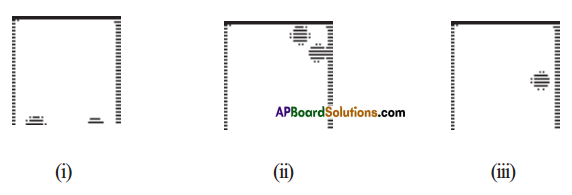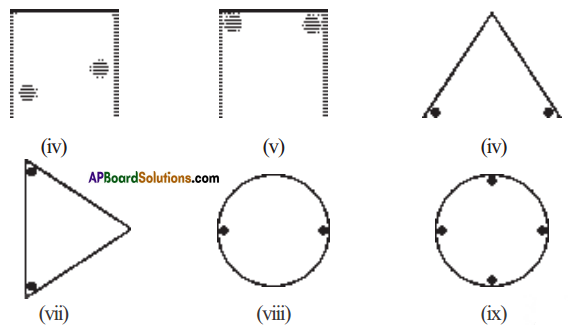Solution: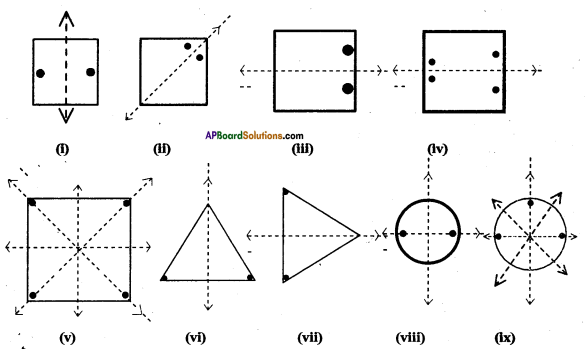Question 2.
Given the line of symmetry, find the other dot.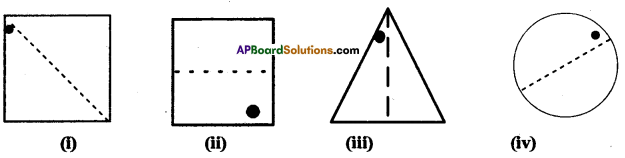Solution: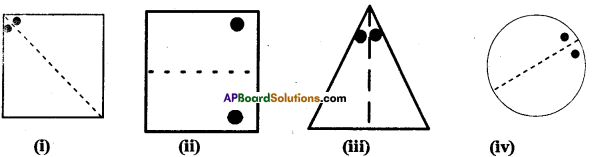Question 3.
In the following incomplete figures, the mirror line (i.e. the line of symmetry) is given as a dotted line. Complete each figure, pcrlorming reflection on the dotted (mirror) line. (You might perhaps place a mirror along the dotted line and look into the mirror for the image). Are you able to recall the name of the figure you complete’?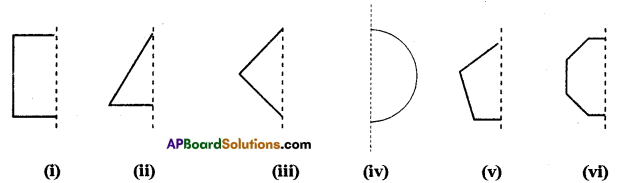Solution: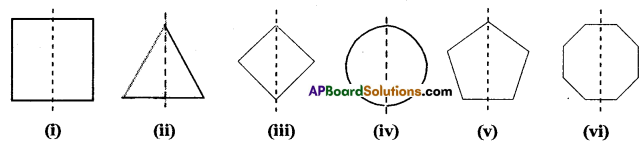Question 4.
State whether the following statements are true or false.
(i) Every closed figure has an axis of symmetry. ( )
(ii) A figure with at least one axis of symmetry is called a symmetric figure. ( )
(in) A regular polygon of 10 sides will have 12 axes of symmetry. ( )
Solution:
(i) False
(ii) True
(iii) False

Question 5.
Draw a square and construct all its axes of symmetry. Measure the angles between each pair of successive axes of symmetry. What do you notice? Does the same rule apply for other regular polygons’?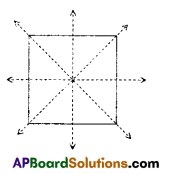Solution:
Angle between successive axes of
Symmetry = 45° = $$\frac{360^{\circ}}{2 \mathrm{n}}$$
This is true for all regular polygons.